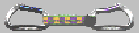# FIST: Fast Industrial-Strength Triangulation of Polygons

The triangulation of a polygon is a basic building block for many graphics applications. For instance, high-speed rendering typically relies on polygonal and curved surfaces being subdivided into triangles that can be handled efficiently by the graphics hardware. Triangulating a polygon also is a fundamental operation in computational geometry, and it has received wide-spread interest over the last two decades. Through a series of improvements, the worst-case complexity of triangulating a polygon has been brought down to linear, achieved by Chazelle's seminal algorithm.

Virtually all published triangulation algorithms assume that the polygon is simple, i.e., that the vertices of the polygon are the only points of the plane that belong to two edges, and that no point of the plane belongs to more than two edges. Obviously, the requirement to be simple prevents a polygon from having self-intersections. However, it also excludes so-called "degenerate" situations, such as an edge passing through another vertex, zero-length edges, and edges that partially overlap. Unfortunately, real-world polygons cannot be assumed to be truly simple polygons that are in general position. Rather, real-world polygons tend to exhibit all types of deficiencies, such as self-intersections, or holes that overlap with the outer boundary.

FIST, my code for fast industrial-strength triangulation, can triangulate a multiply-connected polygonal area (in 2D or 3D) defined by one "outer boundary" (closed polygonal loop) and (possibly) several "holes" (closed polygonal loops or points within the outer boundary). In an ideal world, all polygons lie within one plane, are simple, and do not overlap pairwise. Furthermore, all holes lie strictly within their outer boundary. A recursive nesting of the holes is not supported. The orientations and nesting order of the polygons do not matter; FIST determines which polygon forms the outer boundary and assigns appropriate orientations to all polygons.

For 3D polygons, FIST first projects the 3D vertices onto a plane in order to reduce the 3D triangulation problem to a 2D triangulation problem. As far as vertices that are not co-planar are concerned, this does not constitute a problem provided that the projection does not create deficiencies such as self-intersections. FIST uses a fairly reliable heuristic for finding a decent plane. However, as is well-known, the general problem of projecting or triangulating a general 3D polygon in a "nice" way is provably very difficult from a computational point of view. That is, for a general 3D polygon it is not even clear how to define a "correct" triangulation. Granted, a human often has a good intuition of what a "good" triangulation would be. Experience tells me that the heuristics employed by FIST tend to produce "good" triangulations even for problematic data. However, there is no guarantee that FIST will come up with an ideal plane or triangulation if the vertices are highly not co-planar. (Minor deficiencies introduced by the projection typically still do not result in an "incorrect" triangulation.)

If one or several of the pre-conditions on the input are violated - e.g., if polygons overlap or if they have self-intersections - then FIST applies heuristics in order to succeed with computing a triangulation. The bottom line is that FIST will always come up with a triangulation such that

• every edge of every input polygon belongs to exactly one triangle,
• every edge of a triangle is shared with exactly one other triangle or belongs to exactly one edge of an input polygon,
• every vertex of a triangle is an original vertex of an input polygon,
• the adjacency graph of the triangles is connected.
That is, when viewed in 3D the triangulation computed always forms a surface that interconnects the input polygons. In particular, please note that FIST does not introduce Steiner vertices at points of (self-)intersection of the input polygon(s)!

So, what happens if a polygon contains (self-)intersections? Unfortunately, no universally accepted definition for "triangulation of a polygon" exists if the polygon is allowed to be not simple. Personally, I can think of at least three different definitions which will result in strikingly different triangulations being "correct":

• Treat the 2D polygons as projections of 3D polygons onto a common plane and make sure that the 2D triangulation computed forms a neat triangulation of the surface defined by the 3D polygons once lifted back to 3D. This is what FIST does; please see the images below.
• Insert every point of intersection among the contours as a new Steiner vertex, compute a conforming triangulation of the convex hull of all vertices, and discard those triangles that lie in the "exterior" of the area specified by the polygons, where an appropriate notion of "interior/exterior" has to be defined. This is what a good algorithm for computing constrained Delaunay triangulations will do. However, note that there are at least two different definitions of "interior/exterior" for a polygon that is not simple: one might regard every bounded region of the planar subdivision implied by the polygon(s) as an "interior" region, or one might want to apply winding numbers (or a similar rule) for choosing only a subset of the bounded regions!

Neither of these approaches is trivial to implement, and which one is more desirable depends entirely on the user's needs. In any case, let me clearly state that FIST only supports the first approach, and cannot be extended to support the other approaches! What constitutes a proper triangulation of a polygon that is not simple really is application-dependent.

FIST is based on repeatedly clipping ears of a polygon. The main focus of my work was on designing and implementing an algorithm that is

• completely reliable,
• easy to implement, and
• fast in practice.

The algorithm was implemented in ANSI C, based on floating-point arithmetic. Geometric hashing is used in order to speed up the ear-clipping process in practice. Extensive tests have shown that geometric hashing works well in order to make this approach competitive with (or even faster than) other popular triangulation algorithms that have a better worst-case complexity.

Particular care has been taken in order to make the algorithm reliable. Due to a series of heuristics that serve as a back-up for the standard ear-clipping process if the code detects deficiencies in the input polygon, FIST can handle any type of polygonal input data, be it simple or not. FIST will never crash when applied to polygonal data which has deficiencies. Rather, it will always attempt to generate a meaningful triangulation, and the intuitive "correctness" of the triangulation will gracefully degrade the more the polygon is messed up. Note, though, that FIST will slow down when it has to handle input that has self-intersections.

FIST forms the core of a package for triangulating the faces of 3D polyhedra. FIST can input OBJ files, and can output OBJ and SGO files. A small subset of FIST 1.6 was integrated into Java3D by Sun Microsystems in 1998. However, please note that the current version of FIST is much more powerful than what is provided as part of Java3D. (And even in 1998 the Java-transcribed version of FIST could not rival with FIST concerning both the speed of computation and the quality of the triangulation generated; Sun Microsystems decided to omit important parts of FIST 1.6 from its Java-transcribed version . . .)

I appreciate your interest in FIST. Still, FIST creates quite a bit of email traffic, and you would save yourself and myself time by not asking whether FIST can do anything in addition to what is described above. Let me clearly state that FIST

• cannot handle a 3D point cloud (e.g., to generate a surface based on data obtained from laser scanning);
• cannot handle a 2D point cloud, unless a bounding box of those points is included as input; if you need to triangulate 2D point data then you'd be better off with my Voronoi/Delaunay code "VRONI";
• cannot handle open polygonal curves (unless the curves are transformed into closed zero-area polygons placed inside of a closed polygon or bounding box);
• does not determine points of (self-)intersection of the input contour(s).
Thank you for your understanding of the limitations of FIST.

Very recently, FIST was adapted to run in a coarse-grain parallel mode on multi-core machines. And it was extended with a post-processing to make it compute a constrained Delaunay triangulation; see our CGTA'18 publication referenced below.

Financial support and generous hardware grants for the very initial phase of this project were provided Sun Microsystems, and by NSF Grant CCR-9504192. A good deal of the work for the original version of FIST was carried out while I was with the Computational Geometry Lab of the State University of New York at Stony Brook, Stony Brook, USA. The current version of FIST is the result of a major revision and re-writing of that old code, with the work having been carried out entirely here at the University of Salzburg.

The source code for FIST is available for both academic and commercial use. Indeed, the code has been in wide-spread use, both commercially and within academic research projects. Send me an email and I will let you know the details of how you could use my code. However, in order to avoid any disappointment, let me warn you upfront that FIST has not been released into the public domain!

Recent parts of this research were supported by the Austrian FWF Grants L367-N15 and P25816-N15.

Related publications:

G. Eder, M. Held, P. Palfrader (2018):
"Parallelized Ear Clipping for the Triangulation and Constrained Delaunay Triangulation of Polygons".
Computational Geometry: Theory and Application, 73:15-23, 2018.

M. Held, W. Mann (2011):
"An Experimental Analysis of Floating-Point Versus Exact Arithmetic".
Proc. 23rd Canadian Conf. on Computational Geometry, Toronto, Canada, p. 489--494, Aug 2011.

M. Held (2001):
"FIST: Fast Industrial-Strength Triangulation of Polygons".
Algorithmica 30(4): 563-596, 2001.

The following images show triangulations computed by means of FIST. (Click on an image icon in order to see the full-size image. The full-size images have 800x800 pixels.)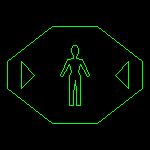A multiply-connected polygonal area with three holes, and its triangulation. The triangulation was computed using "fancy" ear clipping.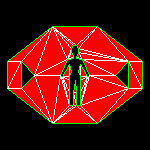A simple polygon, and its Constrained Delaunay triangulation. The CDT was computed using Shewchuk's "Triangle" code. The next row of images shows triangulations computed by "sequential", "random", "sorted", and "fancy" ear clipping. The right-most image shows a triangulation computed by Narkhede and Manocha's implementation of Seidel's randomized algorithm.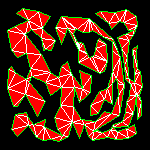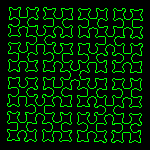A Sierpinski polygon, and its triangulation. The triangulation was computed using "fancy" ear clipping.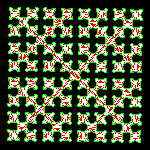A complex star-shaped polygon, and its triangulation. The triangulation was computed using "sorted" ear clipping.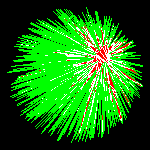A degenerate simply-connected polygonal area, and its triangulation. Note that some of the edges of the polygon overlap. The triangulation was computed using "fancy" ear clipping.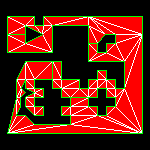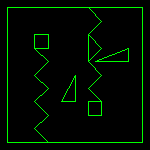A degenerate multiply-connected polygonal area with two holes, and its triangulation. Note that some of the edges of the outer polygon overlap. The triangulation was computed using "fancy" ear clipping.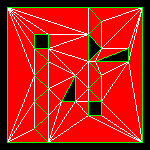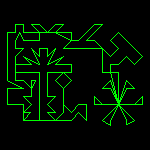A degenerate simply-connected polygonal area, and its triangulation. Note that some of the edges of the polygon (partially) overlap. The triangulation was computed using "fancy" ear clipping. This triangulation contains zero-area triangles (e.g., where edges overlap or where vertices coincide).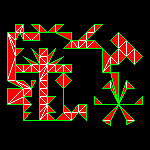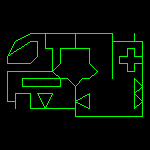A degenerate multiply-connected polygonal area, and its triangulation. Note that some of the edges of the polygon (partially) overlap. The triangulation was computed using "fancy" ear clipping. This triangulation contains zero-area triangles (e.g., where edges overlap or where vertices coincide).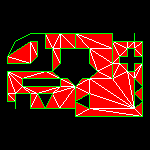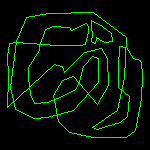A self-overlapping multiply-connected polygon with one hole, and its triangulation. The triangulation was computed using "sorted" ear clipping. Note that a few triangles overlap in 2D! (Please see my comments above on the diverse options for triangulating self-intersecting input prior to telling me that the triangulation is incorrect.)A "multiply-connected" polygonal area with three "not-so-simple" holes, and its triangulation. The triangulation was computed using "sorted" ear clipping. Note that the dual of this triangulation does form a tree. I take votes whether this is the correct triangulation of this area. . . (Again, please see my comments above on the diverse options for triangulating self-intersecting input.)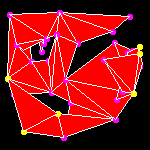Two different views of a curved polygonal surface (in 3D) triangulated by FIST. Note that the polygonal curve does not lie in a plane.Two different views of a polygonal spiral (in 3D) triangulated by FIST. Note that the polygonal curve does not lie in a plane.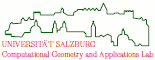file last modified: Wednesday, 08-Aug-2018 10:00:21 CESTCopyright © 2019 Martin Held. All rights reserved.